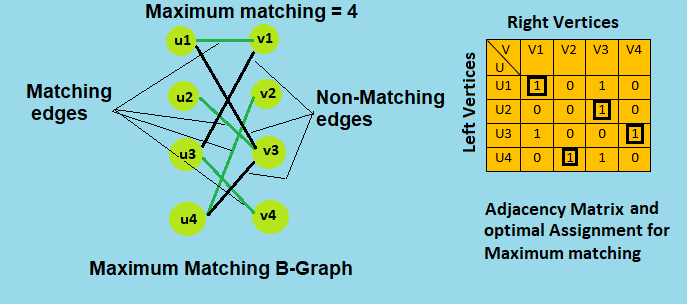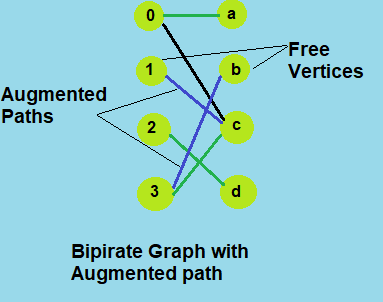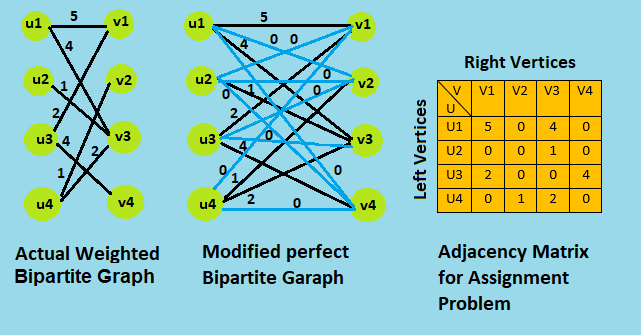×

Search anything:

# Hungarian Maximum Matching Algorithm

#### Algorithms Graph Algorithms maximum matchingGet this book -> Problems on Array: For Interviews and Competitive Programming

The Hungarian maximum matching algorithm, also called the Kuhn-Munkres algorithm, is a O(V3) algorithm that can be used to find maximum-weight matchings in bipartite graphs, which is sometimes called the assignment problem. A bipartite graph can easily be represented by an adjacency matrix, where the weights of edges are the entries.

Bipartite Graph

A bipartite graph (or bigraph) is a graph whose vertices can be divided into two disjoint and independent sets `U` and `V` such that every edge connects a vertex in `U` to one in `V`. Vertex sets `U` and `V` are usually called the parts of the graph. Equivalently, a bipartite graph is a graph that does not contain any odd-length cycles.Matching and Not-Matching edges
Given a matching M, edges that are part of matching are called Matching edges and edges that are not part of M (or connect free nodes) are called Not-Matching edges.

Maximum cardinality matching
Given a bipartite graphs `G = ( V = ( X , Y ) , E )` whose partition has the parts `X` and `Y`, with E denoting the edges of the graph, the goal is to find a matching with as many edges as possible. Equivalently, a matching that covers as many vertices as possible.We now consider weighted bipartite graphs. These are graphs in which each edge `e(i, j)` has a weight, or value, `w(i, j)`. The weight of matching M is the sum of the weights of edges in M, is given by :Now given a bipartite weighted graph G, we have to find a maximum weight matching. Note that, without loss of generality, by adding edges of weight 0, we may assume that G is a complete weighted graph.
To solve this problem, we use Hangarian Algorithm.

Augmenting paths
Given a matching M, an augmenting path is an alternating path that starts from and ends on free vertices. All single edge paths that start and end with free vertices are augmenting paths.The Assignment Problem
Let G be a (complete) weighted bipartite graph. The Assignment problem is to find a max-weight matching in G.
A Perfect Matching is an M in which every vertex is adjacent to some edge in M. A max-weight matching is perfect.
We can convert the bipartite maximum-weighted matching problem to an Assignment Problem, by simply converting the graph to a comlete graph by adding dummy edges of weight `0` and generate the adjacency matrix of the modified graph. We also need same number of vertices in both left and right side for assignment, that can be done by adding dummy vertices.
Then we solve the converted assignment problem by Hungarian method to find maximum-weight matching.# Hangarian Algorithm

The Hungarian algorithm can be executed by manipulating the weights of the bipartite graph in order to find a stable, maximum (or minimum) weight matching. This can be done by finding a feasible labeling of a graph that is perfectly matched, where a perfect matching is denoted as every vertex having exactly one edge of the matching.

Algorithm

Start with any feasible labeling ℓ and some matching M in E.
While M is not perfect repeat the following:

1. Find an augmenting path for Min E;
this increases size of M.
2. If no augmenting path exists,
improve ℓ to ℓ′ such that E ⊂ Eℓ′.
Go to 1.

In each step of the loop we will either be increasing the size of M or E so this process must terminate. Furthermore, when the process terminates, M will be a perfect matching in E for some feasible labeling ℓ. So, by the Kuhn-Munkres theorem (If ℓ is feasible and M is a Perfect matching in E then M is a max-weight matching), M will be a max-weight matching.

In each phase of algorithm, M increases by 1 so there are at most V phases and O(V2) work per phase is done so the total running time is O(V3).

# Implementation

Code in C++

``````// C++ implementation of Hungarian algorithm for maximum matching
// This algorithm works for minimum weight matching
#include <iostream>
#include <algorithm>
#include <vector>
#include <deque>
#include <cassert>
#include <memory>
#include <cstdlib>

#define fo(i, n) for (int i = 0, _n = (n); i < _n; ++i)
#define range(i, a, b) for (int i = (a), _n = (b); i < _n; ++i)

static const int oo = std::numeric_limits<int>::max();
static const int UNMATCHED = -1;

struct WeightedBipartiteEdge
{
int left;
int right;
int cost;

WeightedBipartiteEdge() : left(), right(), cost() {}
WeightedBipartiteEdge(int left, int right, int cost) : left(left), right(right), cost(cost) {}
};

struct LeftEdge
{
int right;
int cost;

LeftEdge() : right(), cost() {}
LeftEdge(int right, int cost) : right(right), cost(cost) {}

const bool operator<(const LeftEdge &otherEdge) const
{
return right < otherEdge.right || (right == otherEdge.right && cost < otherEdge.cost);
}
};

const std::vector<int> hungarianMinimumWeightPerfectMatching(const int n, const std::vector<WeightedBipartiteEdge> allEdges)
{

// Edge lists for each left node.
//const std::unique_ptr< std::vector<LeftEdge> > leftEdges(new std::vector<LeftEdge>[n]);
std::vector< std::vector<LeftEdge> > leftEdges(n);
//region Edge list initialization

// Initialize edge lists for each left node, based on the incoming set of edges.
// While we're at it, we check that every node has at least one associated edge.
// (Note: We filter out the edges that invalidly refer to a node on the left or right outside [0, n).)
{
int leftEdgeCounts[n], rightEdgeCounts[n];
std::fill_n(leftEdgeCounts, n, 0);
std::fill_n(rightEdgeCounts, n, 0);

fo(edgeIndex, allEdges.size())
{
const WeightedBipartiteEdge &edge = allEdges[edgeIndex];
if (edge.left >= 0 && edge.left < n)
{
++leftEdgeCounts[edge.left];
}
if (edge.right >= 0 && edge.right < n)
{
++rightEdgeCounts[edge.right];
}
}

fo(i, n)
{
if (leftEdgeCounts[i] == 0 || rightEdgeCounts[i] == 0)
{
// No matching will be possible.
return std::vector<int>();
}
}

// Probably unnecessary, but reserve the required space for each node, just because?
fo(i, n)
{
leftEdges[i].reserve(leftEdgeCounts[i]);
}
}
// Actually add to the edge lists now.
fo(edgeIndex, allEdges.size())
{
const WeightedBipartiteEdge &edge = allEdges[edgeIndex];
if (edge.left >= 0 && edge.left < n && edge.right >= 0 && edge.right < n)
{
leftEdges[edge.left].push_back(LeftEdge(edge.right, edge.cost));
}
}

// Sort the edge lists, and remove duplicate edges (keep the edge with smallest cost).
fo(i, n)
{
std::vector<LeftEdge> &edges = leftEdges[i];
std::sort(edges.begin(), edges.end());

int edgeCount = 0;
int lastRight = UNMATCHED;
fo(edgeIndex, edges.size())
{
const LeftEdge &edge = edges[edgeIndex];
if (edge.right == lastRight)
{
continue;
}
lastRight = edge.right;
if (edgeIndex != edgeCount)
{
edges[edgeCount] = edge;
}
++edgeCount;
}

edges.resize(edgeCount);
}

//endregion Edge list initialization

// These hold "potentials" for nodes on the left and nodes on the right, which reduce the costs of attached edges.
// We maintain that every reduced cost, cost[i][j] - leftPotential[i] - leftPotential[j], is greater than zero.
int leftPotential[n], rightPotential[n];

//region Node potential initialization

// Here, we seek good initial values for the node potentials.
// Note: We're guaranteed by the above code that at every node on the left and right has at least one edge.

// First, we raise the potentials on the left as high as we can for each node.
// This guarantees each node on the left has at least one "tight" edge.

fo(i, n)
{
const std::vector<LeftEdge> &edges = leftEdges[i];
int smallestEdgeCost = edges.cost;
range(edgeIndex, 1, edges.size())
{
if (edges[edgeIndex].cost < smallestEdgeCost)
{
smallestEdgeCost = edges[edgeIndex].cost;
}
}

// Set node potential to the smallest incident edge cost.
// This is as high as we can take it without creating an edge with zero reduced cost.
leftPotential[i] = smallestEdgeCost;
}

// Second, we raise the potentials on the right as high as we can for each node.
// We do the same as with the left, but this time take into account that costs are reduced
// by the left potentials.
// This guarantees that each node on the right has at least one "tight" edge.

std::fill_n(rightPotential, n, oo);

fo(edgeIndex, allEdges.size())
{
const WeightedBipartiteEdge &edge = allEdges[edgeIndex];
int reducedCost = edge.cost - leftPotential[edge.left];
if (rightPotential[edge.right] > reducedCost)
{
rightPotential[edge.right] = reducedCost;
}
}

//endregion Node potential initialization

// Tracks how many edges for each left node are "tight".
// Following initialization, we maintain the invariant that these are at the start of the node's edge list.
int leftTightEdgesCount[n];
std::fill_n(leftTightEdgesCount, n, 0);

//region Tight edge initialization

// Here we find all tight edges, defined as edges that have zero reduced cost.
// We will be interested in the subgraph induced by the tight edges, so we partition the edge lists for
// each left node accordingly, moving the tight edges to the start.

fo(i, n)
{
std::vector<LeftEdge> &edges = leftEdges[i];
int tightEdgeCount = 0;
fo(edgeIndex, edges.size())
{
const LeftEdge &edge = edges[edgeIndex];
int reducedCost = edge.cost - leftPotential[i] - rightPotential[edge.right];
if (reducedCost == 0)
{
if (edgeIndex != tightEdgeCount)
{
std::swap(edges[tightEdgeCount], edges[edgeIndex]);
}
++tightEdgeCount;
}
}
leftTightEdgesCount[i] = tightEdgeCount;
}

//endregion Tight edge initialization

// Now we're ready to begin the inner loop.

// We maintain an (initially empty) partial matching, in the subgraph of tight edges.
int currentMatchingCardinality = 0;
int leftMatchedTo[n], rightMatchedTo[n];
std::fill_n(leftMatchedTo, n, UNMATCHED);
std::fill_n(rightMatchedTo, n, UNMATCHED);

//region Initial matching (speedup?)

// Because we can, let's make all the trivial matches we can.
fo(i, n)
{
const std::vector<LeftEdge> &edges = leftEdges[i];
fo(edgeIndex, leftTightEdgesCount[i])
{
int j = edges[edgeIndex].right;
if (rightMatchedTo[j] == UNMATCHED)
{
++currentMatchingCardinality;
rightMatchedTo[j] = i;
leftMatchedTo[i] = j;
break;
}
}
}

if (currentMatchingCardinality == n)
{
// Well, that's embarassing. We're already done!
return std::vector<int>(leftMatchedTo, leftMatchedTo + n);
}

//endregion Initial matching (speedup?)

// While an augmenting path exists, we add it to the matching.
// When an augmenting path doesn't exist, we update the potentials so that an edge between the area
// we can reach and the unreachable nodes on the right becomes tight, giving us another edge to explore.
//
// We proceed in this fashion until we can't find more augmenting paths or add edges.
// At that point, we either have a min-weight perfect matching, or no matching exists.

//region Inner loop state variables

// One point of confusion is that we're going to cache the edges between the area we've explored
// that are "almost tight", or rather are the closest to being tight.
// This is necessary to achieve our O(N^3) runtime.
//
// rightMinimumSlack[j] gives the smallest amount of "slack" for an unreached node j on the right,
// considering the edges between j and some node on the left in our explored area.
//
// rightMinimumSlackLeftNode[j] gives the node i with the corresponding edge.
// rightMinimumSlackEdgeIndex[j] gives the edge index for node i.

int rightMinimumSlack[n], rightMinimumSlackLeftNode[n], rightMinimumSlackEdgeIndex[n];

std::deque<int> leftNodeQueue;
bool leftSeen[n];
int rightBacktrack[n];

// Note: the above are all initialized at the start of the loop.

//endregion Inner loop state variables

while (currentMatchingCardinality < n)
{

//region Loop state initialization

// Clear out slack caches.
// Note: We need to clear the nodes so that we can notice when there aren't any edges available.
std::fill_n(rightMinimumSlack, n, oo);
std::fill_n(rightMinimumSlackLeftNode, n, UNMATCHED);

// Clear the queue.
leftNodeQueue.clear();

// Mark everything "unseen".
std::fill_n(leftSeen, n, false);
std::fill_n(rightBacktrack, n, UNMATCHED);

//endregion Loop state initialization

int startingLeftNode = UNMATCHED;

//region Find unmatched starting node

// Find an unmatched left node to search outward from.
// By heuristic, we pick the node with fewest tight edges, giving the BFS an easier time.
{
int minimumTightEdges = oo;
fo(i, n)
{
if (leftMatchedTo[i] == UNMATCHED && leftTightEdgesCount[i] < minimumTightEdges)
{
minimumTightEdges = leftTightEdgesCount[i];
startingLeftNode = i;
}
}
}

//endregion Find unmatched starting node

assert(startingLeftNode != UNMATCHED);

assert(leftNodeQueue.empty());
leftNodeQueue.push_back(startingLeftNode);
leftSeen[startingLeftNode] = true;

int endingRightNode = UNMATCHED;
while (endingRightNode == UNMATCHED)
{

//region BFS until match found or no edges to follow

while (endingRightNode == UNMATCHED && !leftNodeQueue.empty())
{
// Implementation note: this could just as easily be a DFS, but a BFS probably
// has less edge flipping (by my guess), so we're using a BFS.

const int i = leftNodeQueue.front();
leftNodeQueue.pop_front();

std::vector<LeftEdge> &edges = leftEdges[i];
// Note: Some of the edges might not be tight anymore, hence the awful loop.
for (int edgeIndex = 0; edgeIndex < leftTightEdgesCount[i]; ++edgeIndex)
{
const LeftEdge &edge = edges[edgeIndex];
const int j = edge.right;

assert(edge.cost - leftPotential[i] - rightPotential[j] >= 0);
if (edge.cost > leftPotential[i] + rightPotential[j])
{
// This edge is loose now.
--leftTightEdgesCount[i];
std::swap(edges[edgeIndex], edges[leftTightEdgesCount[i]]);
--edgeIndex;
continue;
}

if (rightBacktrack[j] != UNMATCHED)
{
continue;
}

rightBacktrack[j] = i;
int matchedTo = rightMatchedTo[j];
if (matchedTo == UNMATCHED)
{
// Match found. This will terminate the loop.
endingRightNode = j;
}
else if (!leftSeen[matchedTo])
{
// No match found, but a new left node is reachable. Track how we got here and extend BFS queue.
leftSeen[matchedTo] = true;
leftNodeQueue.push_back(matchedTo);
}
}

//region Update cached slack values

// The remaining edges may be to nodes that are unreachable.
// We accordingly update the minimum slackness for nodes on the right.

if (endingRightNode == UNMATCHED)
{
const int potential = leftPotential[i];
range(edgeIndex, leftTightEdgesCount[i], edges.size())
{
const LeftEdge &edge = edges[edgeIndex];
int j = edge.right;

if (rightMatchedTo[j] == UNMATCHED || !leftSeen[rightMatchedTo[j]])
{
// This edge is to a node on the right that we haven't reached yet.

int reducedCost = edge.cost - potential - rightPotential[j];
assert(reducedCost >= 0);

if (reducedCost < rightMinimumSlack[j])
{
// There should be a better way to do this backtracking...
// One array instead of 3. But I can't think of something else. So it goes.
rightMinimumSlack[j] = reducedCost;
rightMinimumSlackLeftNode[j] = i;
rightMinimumSlackEdgeIndex[j] = edgeIndex;
}
}
}
}

//endregion Update cached slack values
}

//endregion BFS until match found or no edges to follow

//region Update node potentials to add edges, if no match found

if (endingRightNode == UNMATCHED)
{
// Out of nodes. Time to update some potentials.
int minimumSlackRightNode = UNMATCHED;

//region Find minimum slack node, or abort if none exists

int minimumSlack = oo;
fo(j, n)
{
if (rightMatchedTo[j] == UNMATCHED || !leftSeen[rightMatchedTo[j]])
{
// This isn't a node reached by our BFS. Update minimum slack.
if (rightMinimumSlack[j] < minimumSlack)
{
minimumSlack = rightMinimumSlack[j];
minimumSlackRightNode = j;
}
}
}

if (minimumSlackRightNode == UNMATCHED || rightMinimumSlackLeftNode[minimumSlackRightNode] == UNMATCHED)
{
// The caches are all empty. There was no option available.
// This means that the node the BFS started at, which is an unmatched left node, cannot reach the
// right - i.e. it will be impossible to find a perfect matching.

return std::vector<int>();
}

//endregion Find minimum slack node, or abort if none exists

assert(minimumSlackRightNode != UNMATCHED);

// Adjust potentials on left and right.
fo(i, n)
{
if (leftSeen[i])
{
leftPotential[i] += minimumSlack;
if (leftMatchedTo[i] != UNMATCHED)
{
rightPotential[leftMatchedTo[i]] -= minimumSlack;
}
}
}

fo(j, n)
{
if (rightMatchedTo[j] == UNMATCHED || !leftSeen[rightMatchedTo[j]])
{
rightMinimumSlack[j] -= minimumSlack;

// If the slack hit zero, then we just found ourselves a new tight edge.
if (rightMinimumSlack[j] == 0)
{
const int i = rightMinimumSlackLeftNode[j];
const int edgeIndex = rightMinimumSlackEdgeIndex[j];

//region Update leftEdges[i] and leftTightEdgesCount[i]

// Move it in the relevant edge list.
if (edgeIndex != leftTightEdgesCount[i])
{
std::vector<LeftEdge> &edges = leftEdges[i];
std::swap(edges[edgeIndex], edges[leftTightEdgesCount[i]]);
}
++leftTightEdgesCount[i];

//endregion Update leftEdges[i] and leftTightEdgesCount[i]

// If we haven't already encountered a match, we follow the edge and update the BFS queue.
// It's possible this edge leads to a match. If so, we'll carry on updating the tight edges,
if (endingRightNode == UNMATCHED)
{
// We're contemplating the consequences of following (i, j), as we do in the BFS above.
rightBacktrack[j] = i;
int matchedTo = rightMatchedTo[j];
if (matchedTo == UNMATCHED)
{
// Match found!
endingRightNode = j;
}
else if (!leftSeen[matchedTo])
{
// No match, but new left node found. Extend BFS queue.
leftSeen[matchedTo] = true;
leftNodeQueue.push_back(matchedTo);
}
}
}
}
}
}

//endregion Update node potentials to add edges, if no match found
}

// At this point, we've found an augmenting path between startingLeftNode and endingRightNode.
// We'll just use the backtracking info to update our match information.

++currentMatchingCardinality;

//region Backtrack and flip augmenting path

{
int currentRightNode = endingRightNode;
while (currentRightNode != UNMATCHED)
{
const int currentLeftNode = rightBacktrack[currentRightNode];
const int nextRightNode = leftMatchedTo[currentLeftNode];

rightMatchedTo[currentRightNode] = currentLeftNode;
leftMatchedTo[currentLeftNode] = currentRightNode;

currentRightNode = nextRightNode;
}
}

//end region Backtrack and flip augmenting path
}

return std::vector<int>(leftMatchedTo, leftMatchedTo + n);
}

int main()
{

int n , e;
std::cin >> n >> e;
std::vector<WeightedBipartiteEdge> edges;
int u, v, w;
for (int i = 0; i < e; ++i)
{
std::cin >> u >> v >> w;
edges.push_back(WeightedBipartiteEdge(u, v, w));
}
std::vector<int> matching ;
matching = hungarianMinimumWeightPerfectMatching(n, edges);

if (matching.empty())
{
std::cout << "=> No matching was found.\n";
}
else
{   std::cout << "Matching found is:\n";
for (int i = 0; i < n; ++i)
{
std::cout << i << " -> " << matching[i] << '\n';
}
}
std::cout << std::endl;
return EXIT_FAILURE;

}
``````

Sample Input and Output
The sample input used is of the bipartite graph shown in the demonstration of the assignment problem.

``````//Input
4 7 // vertices on left and right (same so enter left) and edges
0 0 5 // edges and cost
0 2 4
1 2 1
2 0 2
2 3 4
3 1 1
3 2 2
//Output
Matching found is:
0 -> 0
1 -> 2
2 -> 3
3 -> 1
``````

# Complexity

Time Complexity

• Hungarian Algorithm takes O(V3) time.

Space Complexity

• Hungarian Algorithm takes O(V2) auxiliary space.